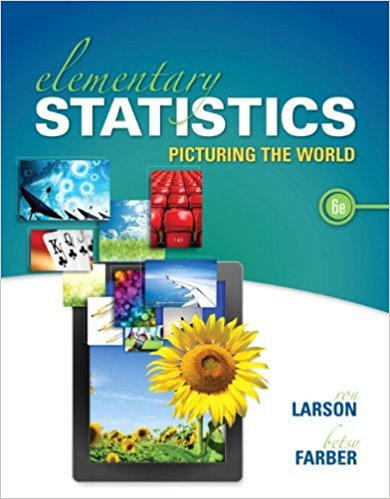×
×

# Solutions for Chapter 10.2: Independence## Full solutions for Elementary Statistics: Picturing the World | 6th Edition

ISBN: 9780321911216Solutions for Chapter 10.2: Independence

Solutions for Chapter 10.2
4 5 0 332 Reviews
29
5
##### ISBN: 9780321911216

This textbook survival guide was created for the textbook: Elementary Statistics: Picturing the World , edition: 6. This expansive textbook survival guide covers the following chapters and their solutions. Elementary Statistics: Picturing the World was written by and is associated to the ISBN: 9780321911216. Chapter 10.2: Independence includes 35 full step-by-step solutions. Since 35 problems in chapter 10.2: Independence have been answered, more than 109243 students have viewed full step-by-step solutions from this chapter.

Key Statistics Terms and definitions covered in this textbook
• `-error (or `-risk)

In hypothesis testing, an error incurred by rejecting a null hypothesis when it is actually true (also called a type I error).

• Alias

In a fractional factorial experiment when certain factor effects cannot be estimated uniquely, they are said to be aliased.

• All possible (subsets) regressions

A method of variable selection in regression that examines all possible subsets of the candidate regressor variables. Eficient computer algorithms have been developed for implementing all possible regressions

• Average

See Arithmetic mean.

• Average run length, or ARL

The average number of samples taken in a process monitoring or inspection scheme until the scheme signals that the process is operating at a level different from the level in which it began.

• Axioms of probability

A set of rules that probabilities deined on a sample space must follow. See Probability

• Bernoulli trials

Sequences of independent trials with only two outcomes, generally called “success” and “failure,” in which the probability of success remains constant.

• Binomial random variable

A discrete random variable that equals the number of successes in a ixed number of Bernoulli trials.

• Bivariate normal distribution

The joint distribution of two normal random variables

• Chance cause

The portion of the variability in a set of observations that is due to only random forces and which cannot be traced to speciic sources, such as operators, materials, or equipment. Also called a common cause.

• Continuity correction.

A correction factor used to improve the approximation to binomial probabilities from a normal distribution.

• Continuous random variable.

A random variable with an interval (either inite or ininite) of real numbers for its range.

• Counting techniques

Formulas used to determine the number of elements in sample spaces and events.

• Covariance matrix

A square matrix that contains the variances and covariances among a set of random variables, say, X1 , X X 2 k , , … . The main diagonal elements of the matrix are the variances of the random variables and the off-diagonal elements are the covariances between Xi and Xj . Also called the variance-covariance matrix. When the random variables are standardized to have unit variances, the covariance matrix becomes the correlation matrix.

• Cumulative distribution function

For a random variable X, the function of X deined as PX x ( ) ? that is used to specify the probability distribution.

• Curvilinear regression

An expression sometimes used for nonlinear regression models or polynomial regression models.

• Deming’s 14 points.

A management philosophy promoted by W. Edwards Deming that emphasizes the importance of change and quality

• Expected value

The expected value of a random variable X is its long-term average or mean value. In the continuous case, the expected value of X is E X xf x dx ( ) = ?? ( ) ? ? where f ( ) x is the density function of the random variable X.

• Frequency distribution

An arrangement of the frequencies of observations in a sample or population according to the values that the observations take on

• Geometric random variable

A discrete random variable that is the number of Bernoulli trials until a success occurs.

×